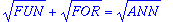## The Alphametic Applet

An alphametic is an arithmetic puzzle in which letters stand for digits. The problem is to find what digits the letters stand for, to make a true equation. Each letter must stand for a different digit, 0 to 9. A number may not start with 0.

For example:
` SIX + SIX + SIX = NINE + NINE`
` 942 + 942 + 942 = 1413 + 1413`

Usually alphametics are solved by hand. With a little algebra and a little patience, it's often not too hard, especially if only addition is involved. Try APPLE + ORANGE = BANANA (two solutions, since L and G are interchangeable), or SEND+MORE=MONEY. Some easy alphametics might even make good math exercises for grade 6 or 7. Multiplication can make it harder, but you may still be able to do it. There is a regular column of alphametics in the Journal of Recreational Mathematics.

With the computer, you can check out lots of possibilities quickly. The challenge is no longer to solve the problem, but to make up a clever one that has a solution. Ideally, there should be just one solution (or some other condition should be added to make it unique), and the words should make sense.

To use the applet, enter the alphametic on the input line and press the "Solve Alphametic" button. Enter it as an equation, just as it appears. You may use +, -, =, * (optional), /, ^ (for integer powers) and parentheses (). Upper and lower case are interchangeable.

Some of my alphametics:

• ``` SUN BURN = RED SKIN ```
• ``` THE BLUE = DANUBE ```
• ``` REASON = IT IS + THERE ```
• ``` FERMAT S = LAST + THEOREM ```
• ``` I HAVE = A DREAM ```
• `````` (however, the applet won't handle the square roots directly)
ROBERT B = ISRAEL